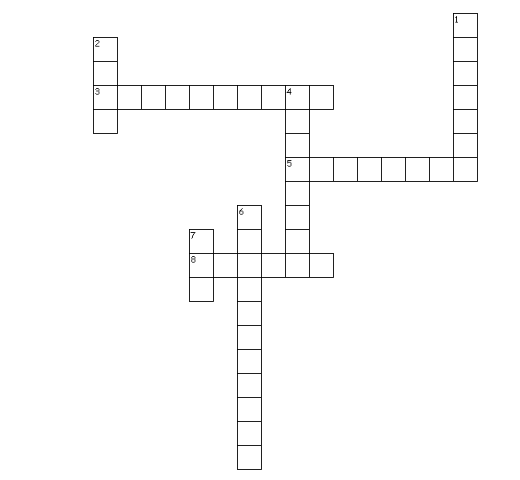# Test Paper for rational Numbers Class 8 maths

In this page we have Test Paper for rational Numbers Class 8 maths . Hope you like them and do not forget to like , social share and comment at the end of the page.
Question 1
True and False statement
i. 0 is a rational number
ii. 0 has multiplicative inverse defined
iii. Subtraction of rational number is commutative.
iv. -1/2 is greater than -2
v. The reciprocal of �1 is -1
vi. 9/6 lies between 1 and 2.
vii. �1 is not the reciprocal of any rational number.
viii. Every whole number is a rational number.
ix. If x and y are two rational numbers such that x > y, then x � y is always a positive rational number.
x. The negative of the negative of any rational number is the number itself

Question 2
Write any 5 rational numbers between 5/6 and 7/8
Question 3
Arrange the below numbers in the ascending order. 5/8 ,1/4 13/16 Question 4
Find the sum of additive inverse and multiplicative inverse of 11?

Question 5
Which of the following is not true?
(a) rational numbers are closed under addition.
(b) rational numbers are closed under subtraction.
(c) rational numbers are closed under multiplication.
(d) rational numbers are closed under division
Question 6
Multiplicative inverse of a negative rational number is
(a) a positive rational number.
(b) a negative rational number.
(c) 0
(d) 1
Question 7
The product of two rational number is 4 , if one of the rational number is 1/5 ,what is the value of other?
Question 8
Write five rational numbers which are smaller than 2
Question 8
Between two given rational numbers, we can find
(a) one and only one rational number.
(b) only two rational numbers.
(c) only ten rational numbers.
(d) infinitely many rational numbers.
Question 9
(a) 11/12
(b) 12/11
(c) -11/12
(d) 0
Question 10
Crossword PuzzleAcross
3. multiplicative inverse is also known as
5. These many rational number exists between any two integers
8. Rational numbers can be represented on a _______ line
Down
1. This property states that when you perform an operation on any two numbers in a certain type of number, the result of the computation is another number in the same type of number
2. It is identity for the addition of rational numbers
4. -a/b is the ____ inverse of a/b
6. The addition and multiplication of whole number ,integers and rational numbers follows this property
7. it is the multiplicative identity for rational numbers